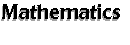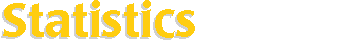[an error occurred while processing this directive] Statistics helps one analyze data collected from say, experiments or questionaires. Then a reasonable model or prediction can be obtained. Software listed here can have a specific purpose or a general purpose, so check it out for yourself. Search SAL: Statistics   (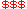Commercial,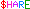Shareware,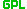GPL) ARfit -- estimation and spectral decomposition of multivariate autoregressive models. |STAT -- data manipulation and analysis programs. AD Model Builder-- rapid development of nonlinear statistical models. CLASP -- a tool for visualizing and statistically analyzing data. Econometrics Toolbox -- statistical estimation, graphics, and data manipulation. ESS-- an Emacs-Lisp interface for interactive statistical programming and data analysis. Elementary Statistical Java Applets and Tools FFE-- GUI front end of ODRPACK for regression. PSPP (originally named Fiasco)-- a program for statistical analysis of sampled data. Genetic LISREL-- a evolutionary program which estimates LISREL models. Goose-- a C++ library dedicated to statistical computation. Gstat-- program for multivariable geostatistical modeling, prediction and simulation. Guide to Basic Laboratory Statistics -- a guide to elementary inferential statistics, including Java applets. ldlas-- ncurses based statistical application. LOCFIT -- local regression and likelihood. MacAnova -- an interactive statistical analysis program. Muac-- a fast (n log(n)) algorithm for the 2D KS test. Multivariate Data Analysis Software -- mostly written in Fortran or C. mwanova-- multi-way analysis of variance of balanced data sets. pasu -- Tcl/Tk based probability and statistics utilities. PEST-- parameter estimation for any model. P-STAT-- an all-in-one statistics software package for social science researcher. ROSTAT -- Fortran program for robust estimation of simple statistics. S-PLUS-- exploratory data analysis and statistical data mining. Shazam-- software for statistical techniques. SGS -- a free geostatistical software tool. Spacestatpack -- Fortran 90 program to estimate large scale spatial autoregressions using maximum likelihood. Spatial Statistics Toolbox -- Matlab toolbox to estimate large scale spatial autoregressions using maximum likelihood. Stata-- a general-purpose statistical package for researchers of all disciplines. statist-- a small, handy and terminal based statistics program. StatistX-- a graphical user interface to statist. TSP-- language for the estimation and simulation of econometric models. UNCERT -- uncertainty analysis and geostatistical software package. ViSta -- visual statistics system. xldlas-- X11 based statistical application. Xlisp-Stat -- extensible statistical computing environment for statistical data analysis. xmstat-- calculates commonly used statistical tests. Also Check Out [B/D] -- an interactive programming language allows analyses of Bayes linear statistical problems. Biostatistics Software -- collection of software for biostatistics research. DATAPLOT -- command driven program for data analysis. Fudgit -- data manipulation, fitting, spline. GAUSS-- a data analysis environment based on the GAUSS matrix language. Glove -- data acquisition, manipulation and analysis program for UNIX/X. Guppi-- plotting data and performing simple statistical manipulations with GUI. LIMDEP-- a complete package for estimating and analyzing econometric models. Mx-- a combination of a matrix algebra interpreter and a numerical optimizer. NNFit -- a non-linear regression program based on multilayered neural network models. Ox -- object-oriented matrix language similar to C/C++. R-- language for statistical calculations which runs most SPlus code. RATS-- a econometrics/time-series analysis software package. StatLib -- a system for distributing statistical software, datasets, and information. XploRe -- an interactive statistical computing environment. Z-IMAGE-- an interactive image analysis package with language similar to statistical package S. Other Links WWW Virtual Library: Statistics http://www.stat.ufl.edu/vlib/statistics.html Statistical Codes for Astronomny and Related Fields http://www.astro.psu.edu/statcodes/ Power analysis software for microcomputers. This page is an attempt to provide an up-to-date list of microcomputer software that can be used to calculate the power of statistical hypothesis tests. http://www.interchg.ubc.ca/cacb/power/ Yahoo! - Science:Mathematics:Statistics:Software http://www.yahoo.com/Science/Mathematics/Statistics/Software/Array-Oriented & Linear Algebra Systems   |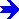Number Theory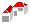SAL Home   |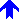Mathematics